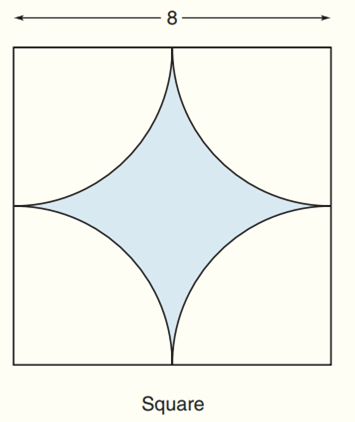Chapter 8.CR, Problem 27CRElementary Geometry For College St...

7th Edition
Alexander + 2 others
ISBN: 9781337614085

Solutions

Chapter
SectionElementary Geometry For College St...

7th Edition
Alexander + 2 others
ISBN: 9781337614085
Textbook Problem

Find the exact areas of the shaded regions in Exercises 27 to 31.To determine

To find:

The exact areas of the shaded regions.

Explanation

Theorem:

The area A of a square whose sides are each of length s is given by A=s2

Calculation:

Sketch the following diagram:

The side length (s) = 8

Radius (r) = 4

The area of quarter circle = 14πr2

The area of shaded region = Area of square - 4×area of quarter circle=

Still sussing out bartleby?

Check out a sample textbook solution.

See a sample solution

The Solution to Your Study Problems

Bartleby provides explanations to thousands of textbook problems written by our experts, many with advanced degrees!

Get Started

Find more solutions based on key concepts